# Torsion submodule

Jump to: navigation, search

Letbe an associative ring with unit, anda left-module. The torsion subgroup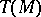is defined as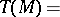Here a regular elementis an element that is not a zero divisor (neither left nor right).

Ifis left Ore (cf. below and Associative rings and algebras), thenis a submodule of, called the torsion submodule. A moduleis torsion free if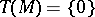. A module is torsion if.

Quite generally, a torsion theory for an Abelian categoryis a pairof subclasses of the objects of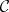such thatfor all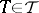,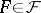and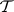and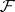are maximal with this property, i.e. if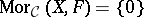for all, then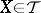, and iffor all, then.

The torsion submodules and torsion-free submodules of a left Ore ringfrom a torsion theory for the category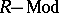of left-modules.

A radical onis a left-exact functorsuch that for all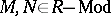,

i)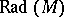is a submodule of;

ii)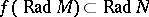for all; more precisely,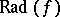is the restriction ofto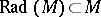.

iii)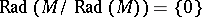.

A radical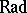is a torsion radical or hereditary radical if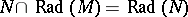for each submoduleof a module. A torsion radicaldefines a torsion theory forwith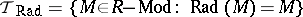,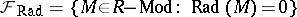. All torsion theories forarise this way.

A left denominator set ofis a submonoidof(i.e.and) such that:

a) (the left Ore condition) for all,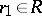there are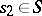,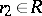such that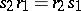;

b) iffor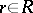,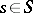, then there is an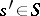with.

If the set of all regular elements ofis a left denominator set, thenis called left Ore. A left denominator set is also called a left Ore set.

A left denominator setdefines a torsion theoryforby the associated radical functor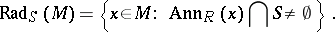This illustrates the links between torsion theories and (non-commutative) localization (theories). For much more about this theme cf. [a1], [a2], [a3], cf. also Fractions, ring of.